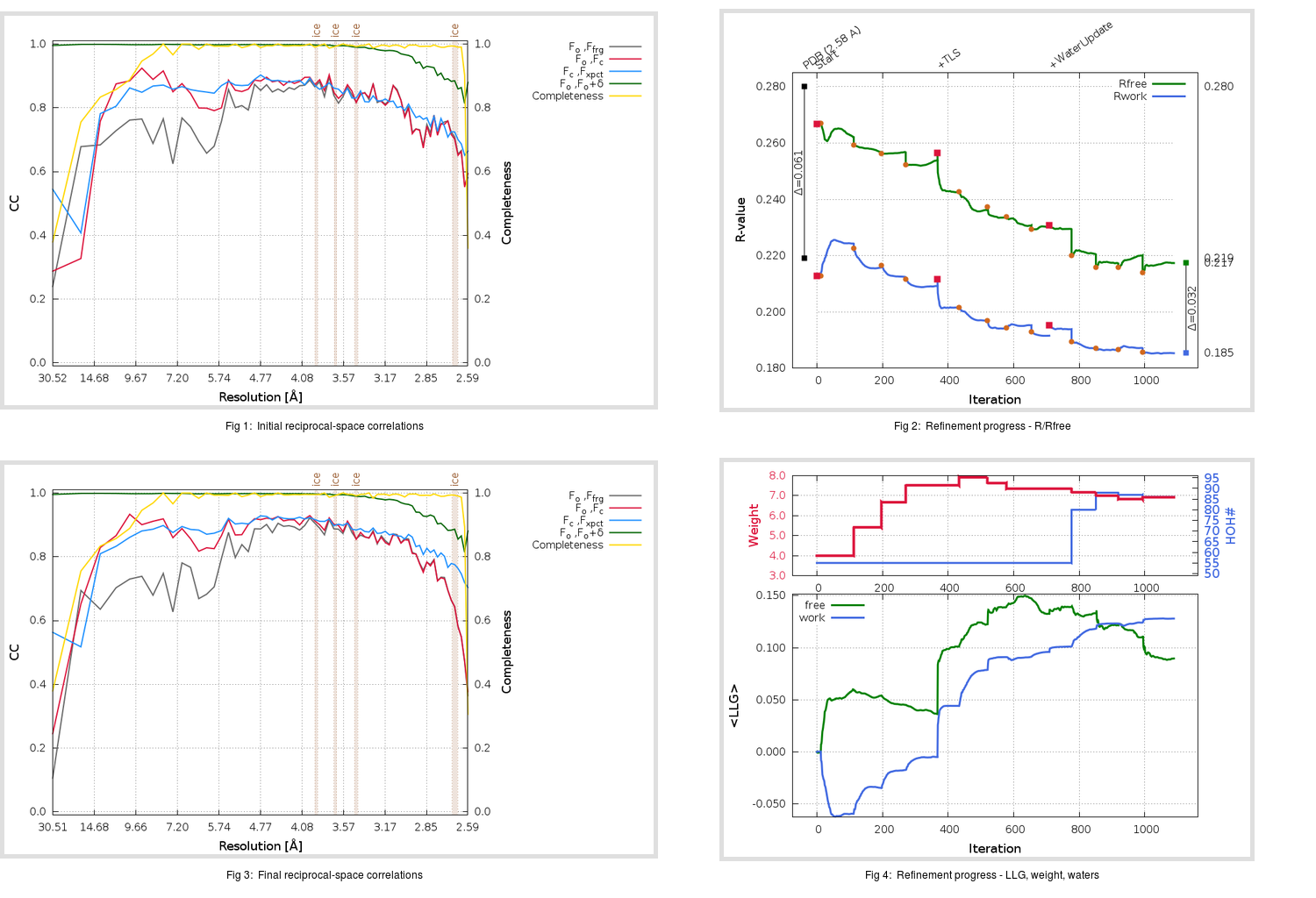Content:

## Deposited

` `
 Date deposited Date data collection Resolution R, Rfree 20110629 20090720 2.58 0.2150 0.2800

Molprobity (CCP4 7.0 version) summary:

```Ramachandran outliers =   1.31 %
favored =  89.87 %
Rotamer outliers      =  11.24 %
C-beta deviations     =     1
Clashscore            =  17.18
RMS(bonds)            =   0.0175
RMS(angles)           =   1.68
MolProbity score      =   3.09
Resolution            =   2.58
R-work                =   0.2150
R-free                =   0.2800
```

```Number of waters      =    55

<B> (all atoms) =   56.45 ( sd =   13.08 ) for       2469 non-hydrogen atoms
<B>   (protein) =   56.41 ( sd =   13.01 ) for       2398 non-hydrogen atoms
<B>     (water) =   51.82 ( sd =    8.57 ) for         55 non-hydrogen atoms
<B>    (others) =   78.67 ( sd =   14.65 ) for         16 non-hydrogen atoms

B min/max       (all non-hydrogen atoms) =   27.29 /   95.62
B min/max   (protein non-hydrogen atoms) =   27.29 /   94.92
B min/max     (water non-hydrogen atoms) =   32.94 /   68.54
B min/max     (other non-hydrogen atoms) =   59.51 /   95.62
```

## BUSTER (re-)refinement

` `

Molprobity (CCP4 7.0 version) summary:

```Ramachandran outliers =   0.00 %
favored =  97.06 %
Rotamer outliers      =   6.74 %
C-beta deviations     =     1
Clashscore            =   5.66
RMS(bonds)            =   0.0115
RMS(angles)           =   1.59
MolProbity score      =   2.10
Resolution            =   2.58
R-work                =   0.1854
R-free                =   0.2174
```

```Number of waters      =    86

<B> (all atoms) =   72.16 ( sd =   16.51 ) for       2500 non-hydrogen atoms
<B>   (protein) =   71.92 ( sd =   16.46 ) for       2398 non-hydrogen atoms
<B>     (water) =   73.58 ( sd =   14.30 ) for         86 non-hydrogen atoms
<B>    (others) =  100.64 ( sd =    5.93 ) for         16 non-hydrogen atoms

B min/max       (all non-hydrogen atoms) =   43.82 /  127.87
B min/max   (protein non-hydrogen atoms) =   43.82 /  127.87
B min/max     (water non-hydrogen atoms) =   47.15 /  110.34
B min/max     (other non-hydrogen atoms) =   92.96 /  111.22
```

Refinement progression:Results:

` `
 File Remark 3SNC_aB_refine.01_03_refine.pdb.gz exact refinement commands are in header 3SNC_aB_refine.01_03_refine.mtz.gz including original deposited data and several re-refinement map coefficients 3SNC_aB_refine.01_03_BUSTER_model.cif.gz including any non-standard compound restraints 3SNC_aB_refine.01_03_BUSTER_refln.cif.gz